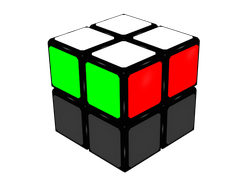## 1. Introduction

This is a LBL (Layer By Layer) method, and the aim is to place correctly one layer before solving the next one. This method is divided into three parts:

1. FL: First Layer. Here, the four stickers of the same colour are joined in the same face, while placing correctly the pieces of this layer.
2. OLL: Orientation of the Last Layer. The aim is to make the opposite face contains just one colour. In this method seven different algorithms are used for this step.
3. PLL: Permutation of the Last Layer. Here, we have to move the pieces of the last layer (while keeping a single colour of the face) until the cube is solved. We will use four algorithms.

As an example to explain this, the white face will be completed first, and the yellow face in the second part. However, all these rules can be applied to no matter what couple of opposite colours of the cube.

## 2. FL (First Layer)

Here, the aim is to put the same colour stickers together in one face, while placing correctly the pieces of this layer (see Figure 1). Although in this guide this part will be explained in a very methodical way, it is actually very intuitive and practice is much more important than memorizing.Figure 1a: Final situation of the cube after FL step. Green and red faces.Figure 1b: Final situation of the cube after FL step. Blue and orange faces.

Table 1 shows the different options when we need a piece on the right of the cube, and Table 2, the options when we need a piece on the left. All the movements can be learnt, or you could use the first five algorithms or the last five, because we can easily pass from a case of the first Table to a case of the second one, just by turning the whole cube. Table 1 will be easier for right-handed people, and Table 2, for left-handed people.

Table 1: Algorithms of the 1st part (FL), with a piece to place on the right

Case

Figure

Algorithms

1

R'D'R

2

D'R'DR

3

R'D'RDR'D'R

4

R'DR - D'R'DR

After the first three movements,

we arrive to case 2.

5

R'DRD2R'D'R

Table 1: Algorithms of the 1st part (FL), with a piece to place on the left

Case

Figure

Algorithms

1

LDL'

2

DLD'L'

3

LDL'D'LDL'

4

LD'L' - DLD'L'

After the first three movements,

we arrive to case 2.

5

LD'L'D2LDL'